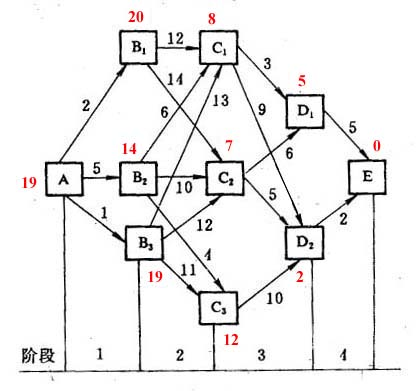# dynamic programming

20世纪50年代初美国数学家R.E.Bellman等人在研究多阶段决策过程(multistep decision process)的优化问题时，提出了著名的最优化原理(principle of optimality)，把多阶段过程转化为一系列单阶段问题，利用各阶段之间的关系，逐个求解，创立了解决这类过程优化问题的新方法——动态规划。

1957年出版了他的名著《Dynamic Programming》，这是该领域的第一本著作。

# 动态规划与递归

## 啥叫「自顶向下」？

int Fibonacci(size_t n)
{
if(n == 1 || n == 2)
{
return n;
}
return Fibonacci1(n-1) + Fibonacci1(n-2);
}


ps: 大家应该还记得，递归的实现看起来很优雅，实际上有很大的限制。计算的数值稍微大一点，基本上是算不出来的。

## 啥叫「自底向上」？

// 动态规划法计算斐波那契数列
int fib(int N) {
vector<int> dp(N + 1, 0);
dp = dp = 1;
for (int i = 3; i <= N; i++)
dp[i] = dp[i - 1] + dp[i - 2];
return dp[N];
}


# 便于理解的例子

## 题目

7
3 8
8 1 0
2 7 4 4
4 5 2 6 5


## 递归解法

class Solution{

public int getMax(){
int MAX = 101;
int[][] D = new int[MAX][MAX];   //存储数字三角形
int n;              //n表示层数
int i = 0; int j = 0;
int maxSum = getMaxSum(D,n,i,j);
return maxSum;
}

public int getMaxSum(int[][] D,int n,int i,int j){
if(i == n){
return D[i][j];
}
int x = getMaxSum(D,n,i+1,j);
int y = getMaxSum(D,n,i+1,j+1);
return Math.max(x,y)+D[i][j];
}
}


### 重复计算

7
3 8
8 1(2) 0
2 7(3) 4(3) 4
4 5(4) 2(6) 6(4) 5


ps: 这里就是动态规划最核心的思想。

## 动态规划解法

for(int i = n-1; i >= 1; i--) {
for(int j = 1; j <= i; j++>) {
maxSum[i][j] = Math.max(maxSum[i+1][j], maxSum[i+1][j+1]);
}
}


# 分类

## 概念引入# 参考资料

【算法复习】动态规划

https://www.jianshu.com/p/40064cb0d5f3